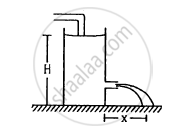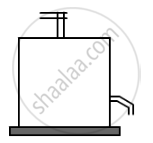Department of Pre-University Education, KarnatakaPUC Karnataka Science Class 11
Advertisement Remove all ads

# Water Level is Maintained in a Cylindrical Vessel up to a Fixed Height H. the Vessel is Kept on a Horizontal Plane. - Physics

Sum

Water level is maintained in a cylindrical vessel up to a fixed height H. The vessel is kept on a horizontal plane. At what height above the bottom should a hole be made in the vessel so that the water stream coming out of the hole strikes the horizontal plane at the greatest distance from the vessel.Advertisement Remove all ads

#### Solution

It is given that H is the height of the cylindrical vessel.Now, let h be the height of the hole from the surface of the tank.
The velocity of water $\left( v \right)$ is given by
$v = \sqrt{2g(H - h)}$
Also, let t be the time of flight.
Now,

$t = \sqrt{\frac{2h}{g}}$

Let x be the maximum horizontal distance .

$\therefore x = v \times t$

$= \sqrt{2g(H - h)} \times \sqrt{\frac{2h}{g}}$

$= \sqrt{4(Hh - h^2 )}$

For x to be maximum,

$\frac{d}{dh}\left( Hh - h^2 \right) = 0$

$\Rightarrow 0 = H - 2h$

$\Rightarrow h = \frac{H}{2}$

Is there an error in this question or solution?
Advertisement Remove all ads

#### APPEARS IN

HC Verma Class 11, 12 Concepts of Physics 1
Chapter 13 Fluid Mechanics
Q 35 | Page 275
Advertisement Remove all ads

#### Video TutorialsVIEW ALL 

Advertisement Remove all ads
Share
Notifications

View all notifications

Forgot password?